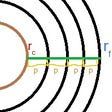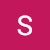# What is an Algorithm?

In computer science, whenever we want to solve some computational problem then we define a set of steps that need to be followed to solve that problem. These steps are collectively known as an algorithm.

Designing algorithms is about making decisions and decisions have tradeoffs. You might want to optimize to complete the task:

• in the shortest time
• using the least amount of space

This where complexity comes in, where you want to answer the following questions:

1. How do you know if your algorithm is good?
2. How do you compare algorithms?

So we can break this down to two concepts:

• Time Complexity -> How long does the algorithm take
• Space Complexity -> How much space does the algorithm use

The less complex we make the algorithm the better!

# An example of an algorithm that may be more time efficient: Time Efficient Grocery Shopping

1. Drive a large car to the grocery store.
2. Buy everything that you need.

So following this algorithm saves time, but requires you to have a large car. This means lower time complexity and higher space complexity

# An example of an algorithm that may be more space efficient: Space Efficient Grocery Shopping

1. Walk to the grocery store with a tote bag.
3. Walk home and drop off the item.
4. Repeat until done.

Following this algorithm takes a long time, but does not require a car. This means higher time complexity and lower space complexity.

# Introducing, Big O Notation

Big O notation (also known as asymptotic notation) is simply a mathematical notation we can use to measure the performance of a function.

# Time Complexity

Constant time, O(1)

Let’s take a look at a very simple function.

public void print(s: String){

System.out.println(s)

}

No matter what the size of the input, this function will always take the same amount of time. We deduce this by the fact that there is always only going to be one “step”.

void print(List<Int> list){

for(int i=0;i<list.size();i++){

for (int j=0;j<list.size();j++){

System.out.println(“i2”)

}

}

}

This function will run in O(n²) or what is known as quadratic time. As we have a nested loop, our run-time will increase exponentially depending on the input. For example, if we have a list with 4 elements, we’d need to print 4 items 4 different times — which equates to 4²;

# Space Complexity

Space complexity is used to classify how much memory a given function will take during run-time. Unlike time complexity, space complexity is much easier to classify, we only need to look at the size of any variables we allocate.

--

--

--

## More from Kotasaisrikanth

Love podcasts or audiobooks? Learn on the go with our new app.

## The Toilet Paper Theorem: The Mathematics behind An Ordinary Roll## Obvious Adams — Quotidian — 386## What is Discrete Math ?## Mathematics and Statistics behind Machine Learning — PART 2## Parallax error — 2## Basics of vectors## What’s Entropy? (Part-II)## Green Energy Revolution or Evolution?## 2444 km from the glory.## K-Means Clustering and geometric mean and standard deviation for the area of 100 Murraya koenigii…